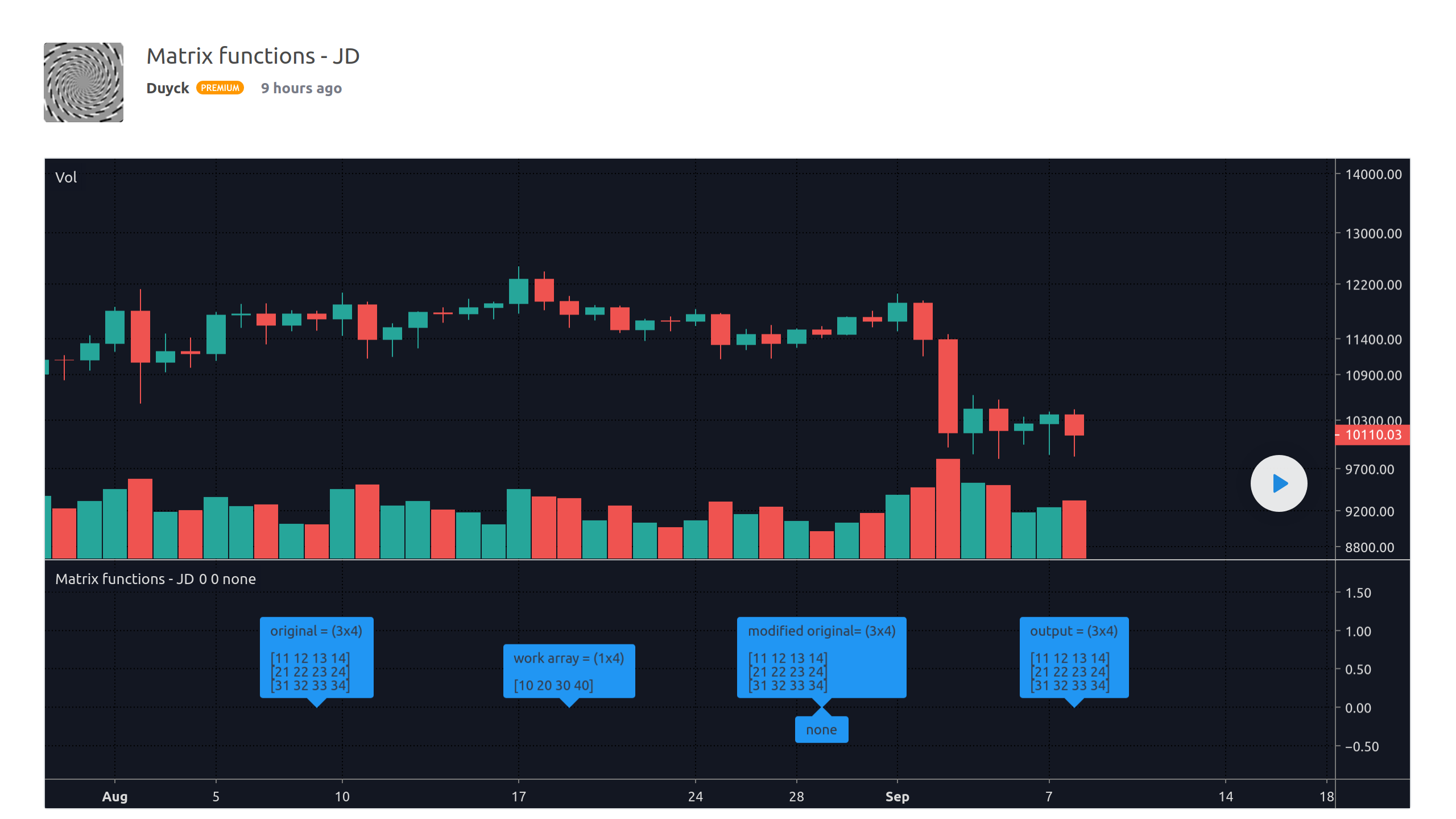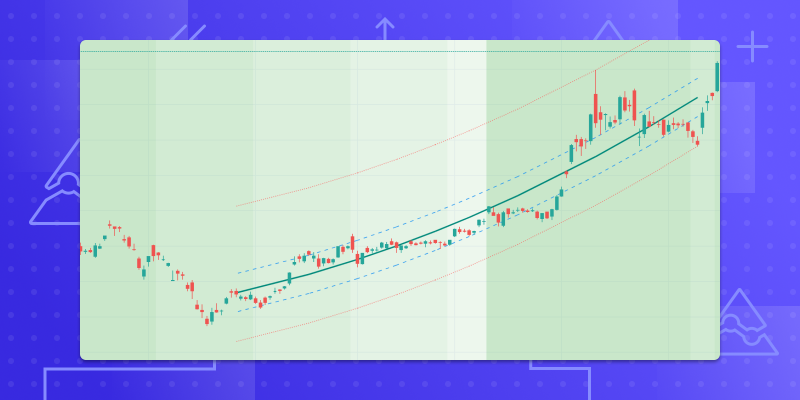September 10, 2020
Pine現在可以使用陣列

Pine腳本現在可以使用強大的新陣列功能來建構自訂數據集。陣列允許程式人員在包含以下類型之一的元件的一維資料結構中進行填充、管理、和計算，從而顯著擴展了Pine的建模功能：float, int, bool, or color

`levels = array.new_float(size = 3, initial_value = na)`

```//@version=4
study("Price Avg Of Increasing Volume Bars", "", true)
i_levels = input(20,   "Levels")
i_src    = input(hlc3, "Source")

// Initialize array of user-selected size with `na` values.
var levels = array.new_float(i_levels)

if rising(volume, 1)
// Remove the oldest level from the beginning of the array.
array.shift(levels)

// Add the new level to the end of the array.
array.push(levels, i_src)

// Calculate the avg of levels in the array, excluding any `na` values.
level = array.avg(levels)
plot(level, "Level", close > level ? color.lime : color.fuchsia)```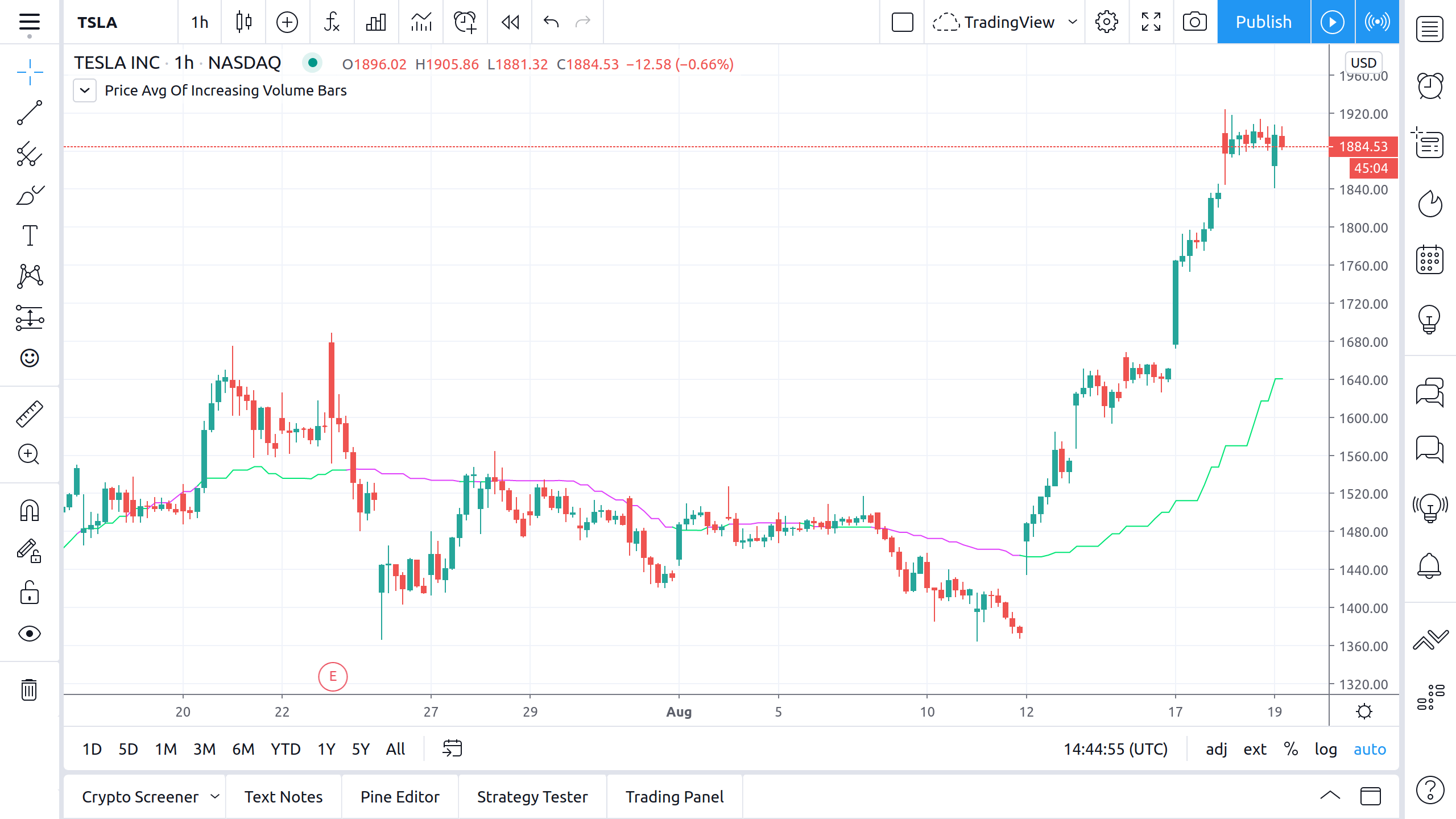Pine用戶手冊的陣列章節將幫助您入門。另外，請參閱PineCoders在這些腳本中發揮作用的陣列，他們在測試陣列方面為我們提供了最大幫助，我們由衷地感謝：RicardoSantosDuyck

Graph style – 4th Dimension RSI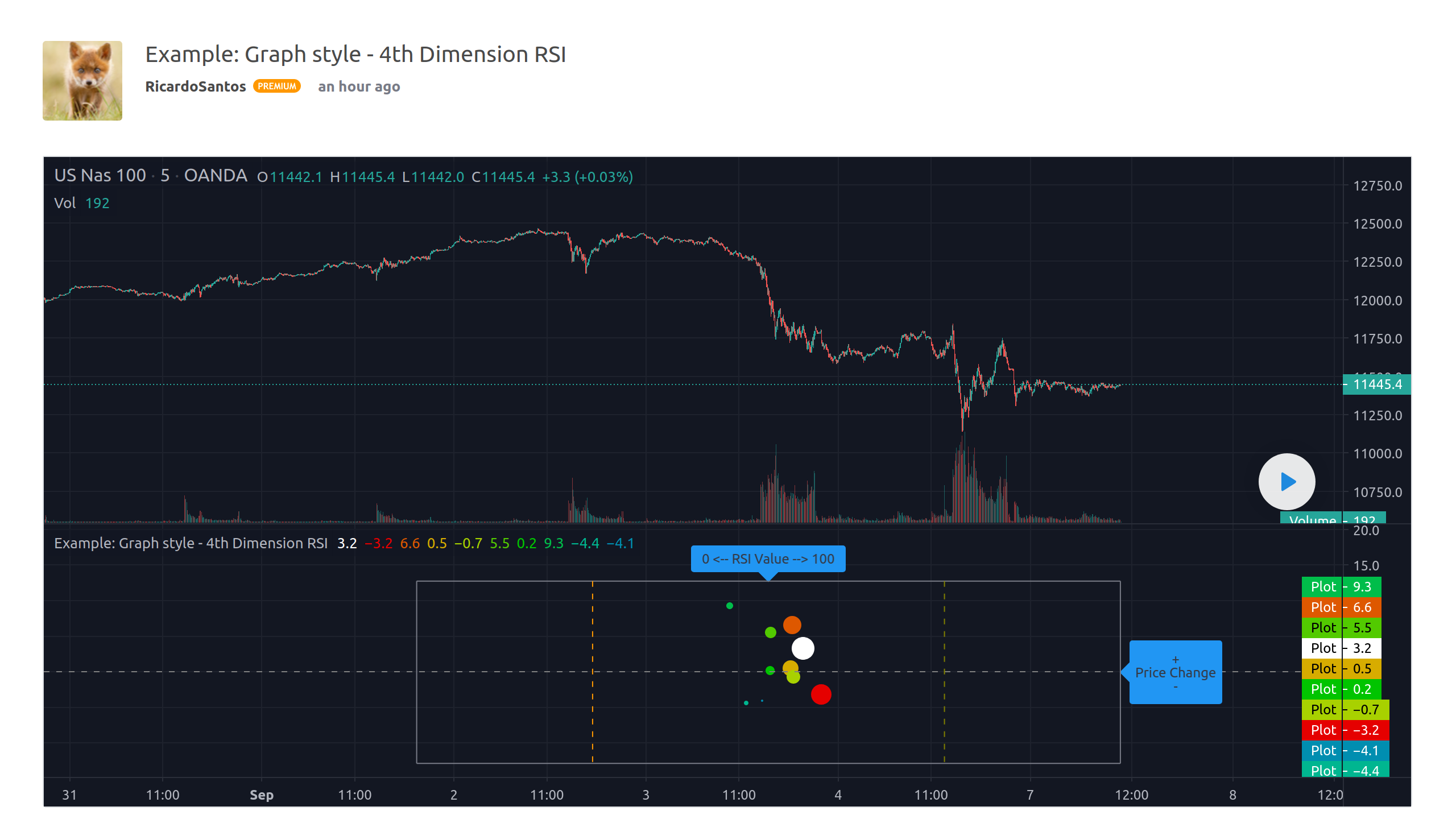Function – Multi Dimension IndexerFunction – K-Means Clustering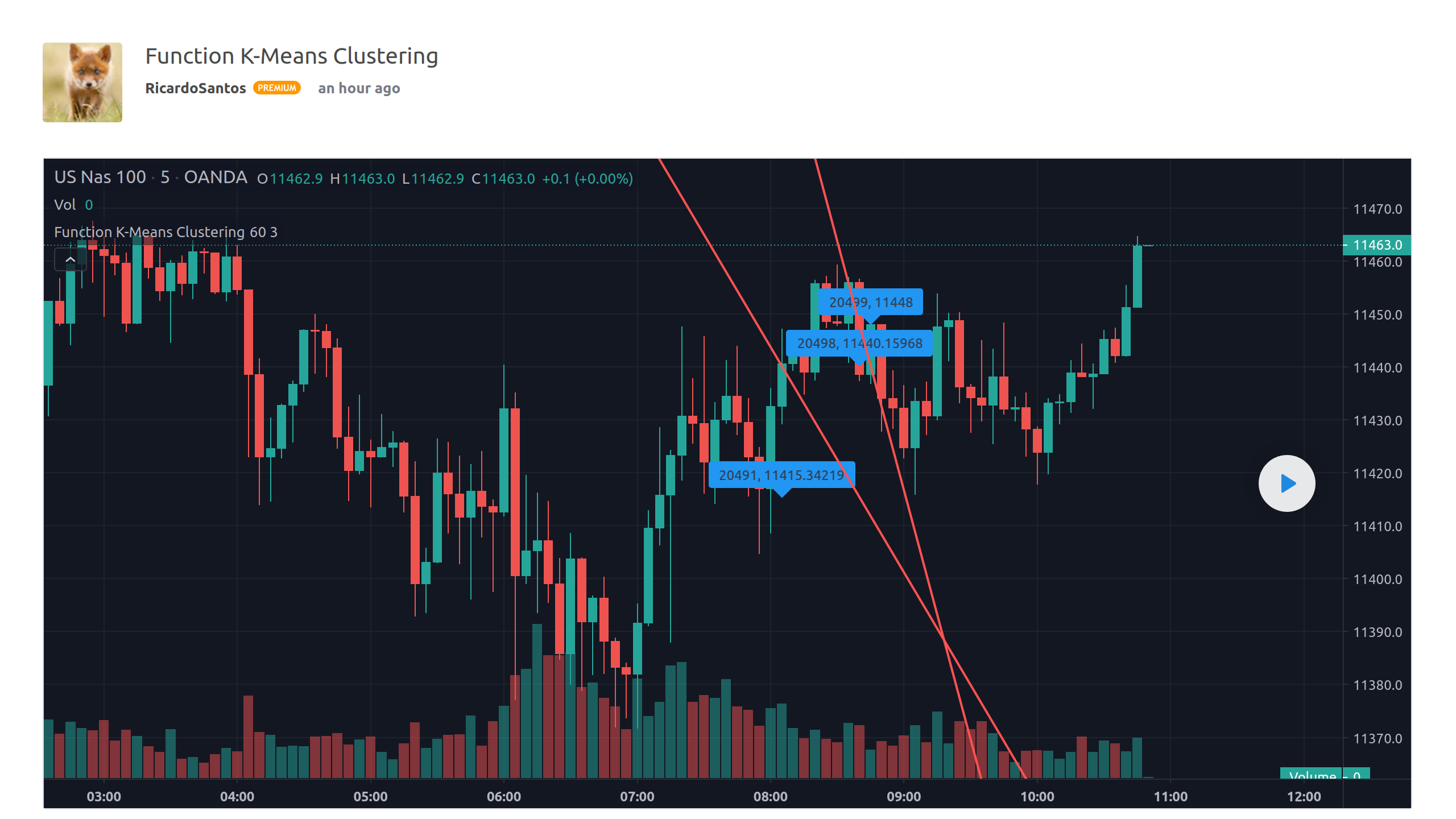Function – Linear RegressionFunction – Polynomial Regression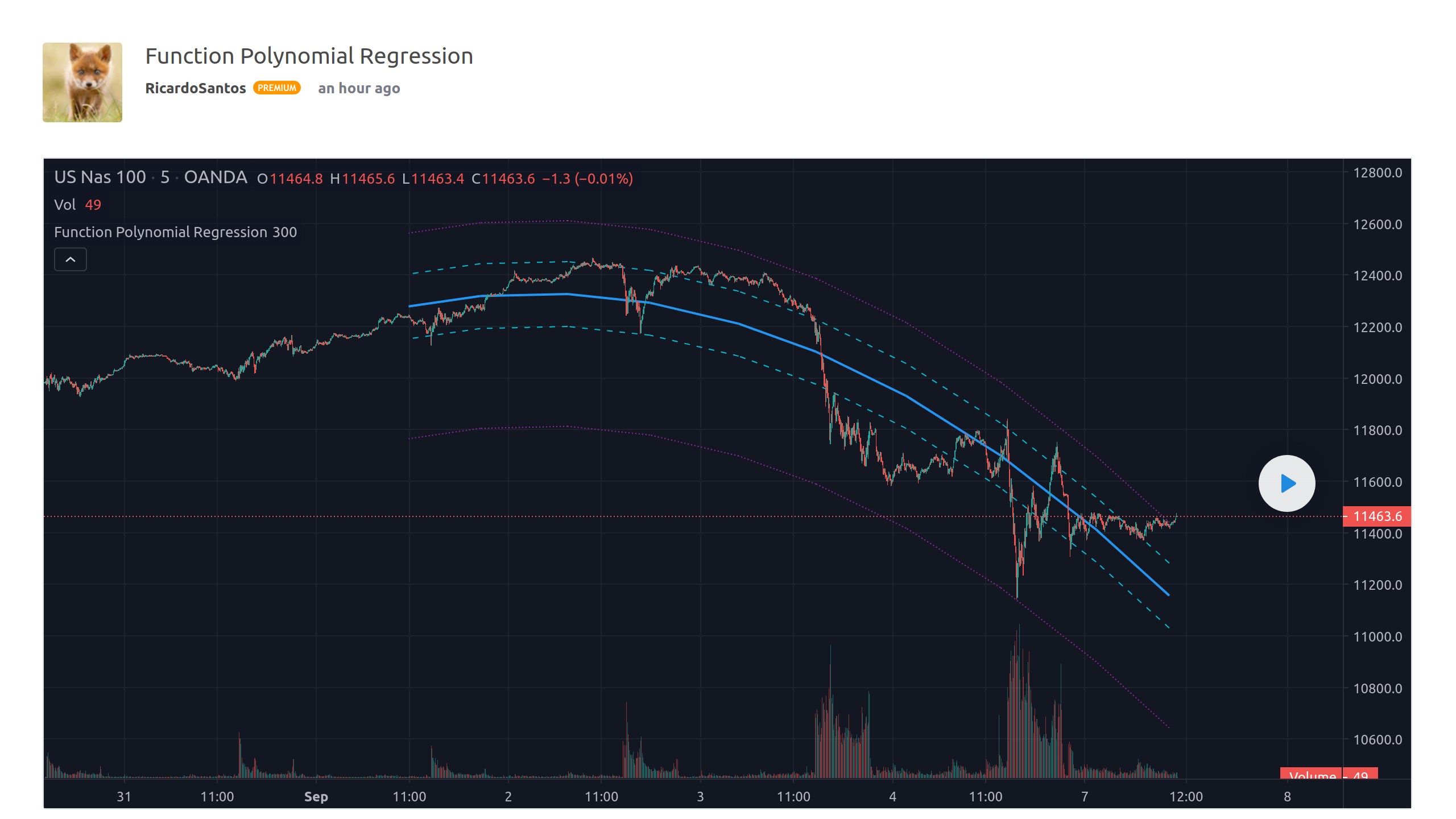Trendlines – JD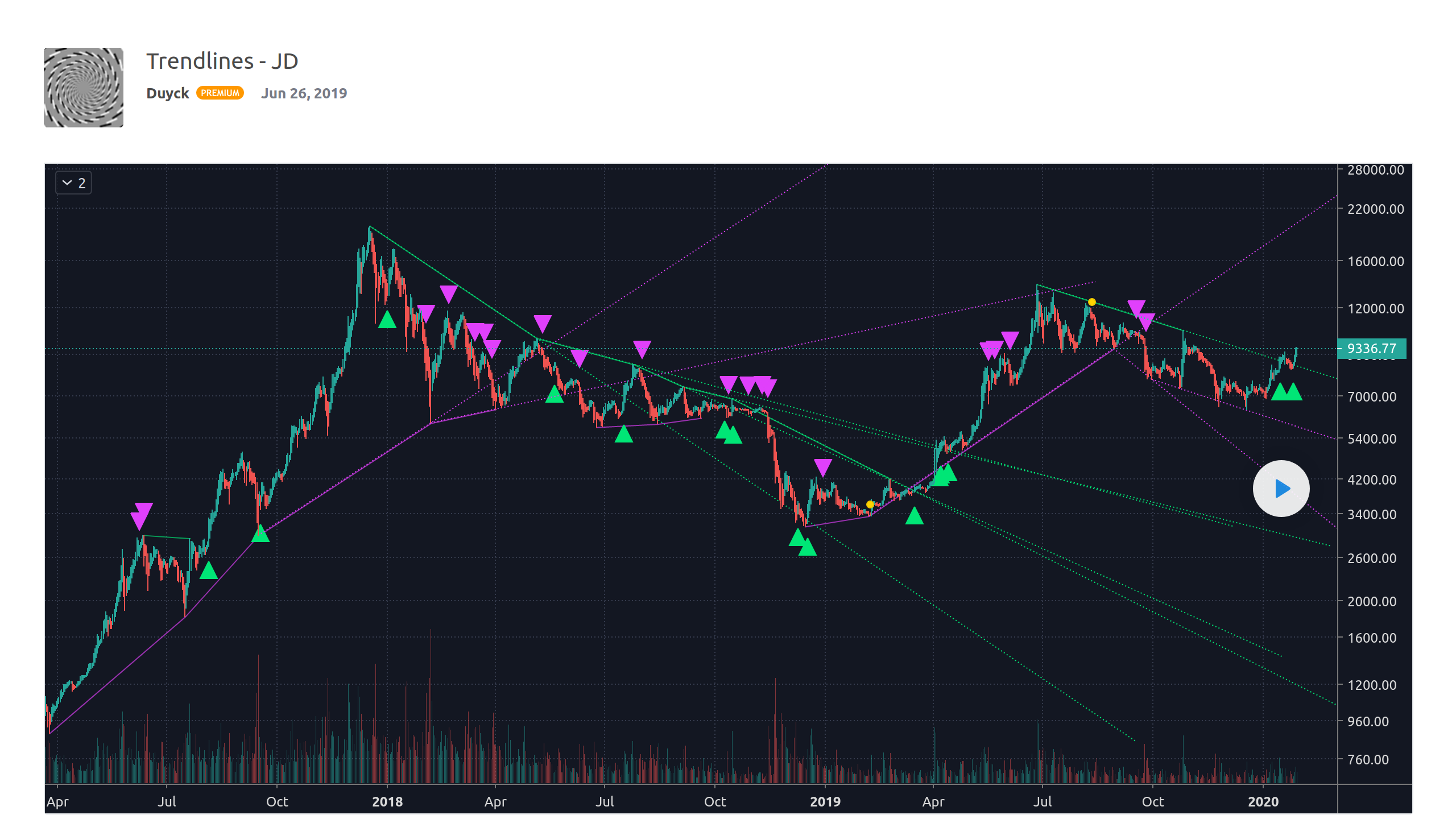Average Pivot Range – JDMatrix functions – JD